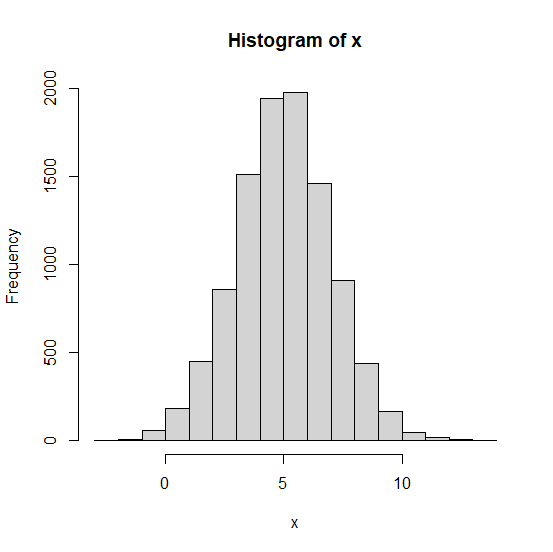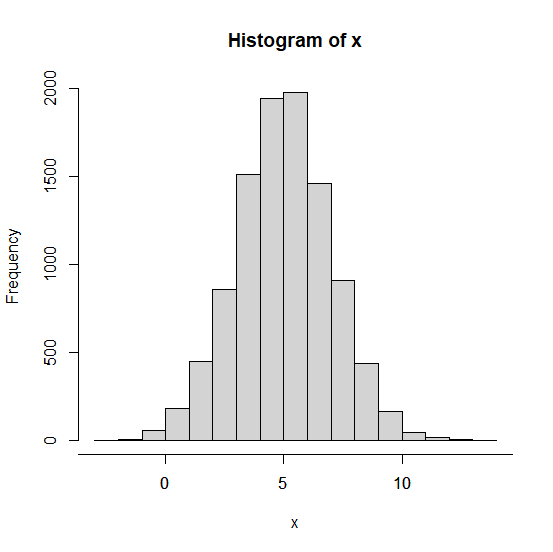# How to increase the X-axis length of histogram in base R?

To increase the X-axis length of histogram in base R, we can use the axis function. The most important thing to remember while using the axis function is mentioning the appropriate sequence based on the values in the vector or in the column of the data frame if a data frame is used.

## Example

Live Demo

x<−rnorm(10000,5,2)
hist(x)

## OutputIncreasing the X-axis length of histogram −

## Example

axis(1,at=seq(−5,15,by=5))

## Output